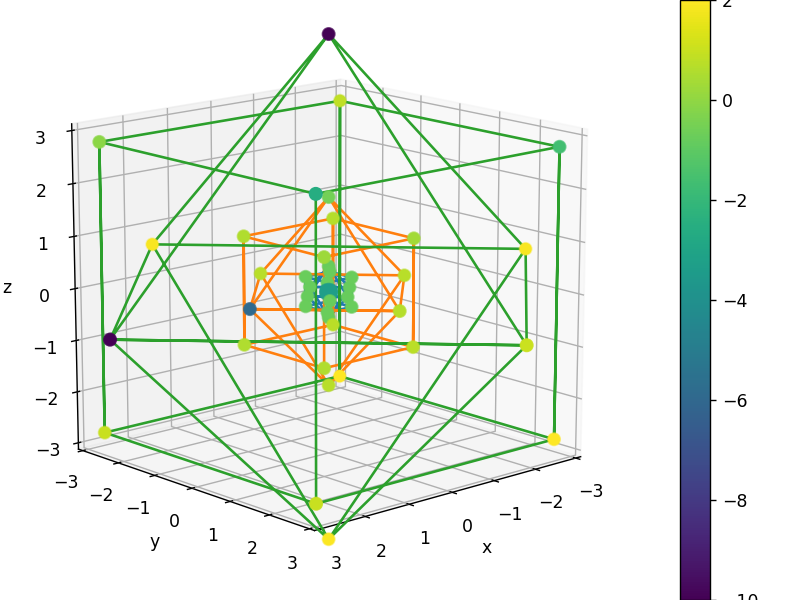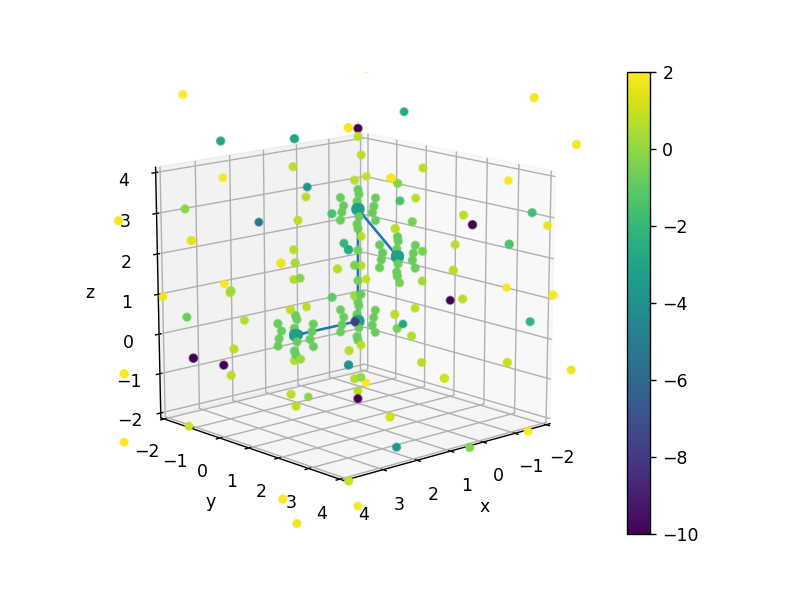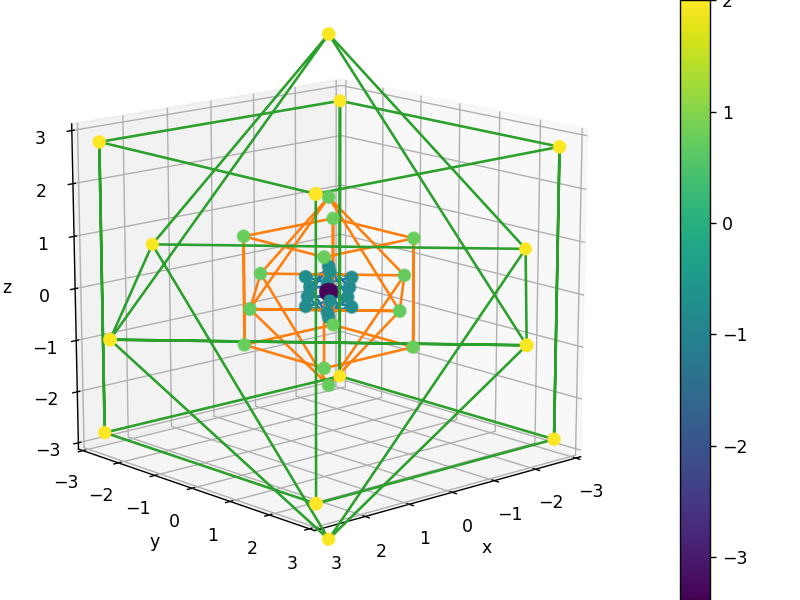5. DFT 格点¶

:
%matplotlib notebook

import numpy as np
import time
from pyscf import gto, lib, dft
from functools import partial

from pyxdh.Utilities.test_molecules import Mol_H2O2
import matplotlib.pyplot as plt
from mpl_toolkits.mplot3d import Axes3D

np.set_printoptions(5, suppress=True, linewidth=120)
np.einsum = partial(np.einsum, optimize=["greedy", 1024 ** 3 * 2 / 8])

5.1. 数值积分概念¶

$\sin(x) , \quad x \in \left[ 0, \pi \right]$

$\int_0^\pi \sin(x) \, \mathrm{d} x = \cos(x) |_{x = 0}^\pi = 2$

$\int_0^\pi \sin(x) \, \mathrm{d} x \sim \sum_{g = 0}^\frac{\pi}{\Delta x} \sin(g \Delta x) \Delta x$

$\int_0^\pi \sin(x) \, \mathrm{d} x \sim \sum_g \sin(x_g) \Delta x$

:
Delta_x = 0.00001
x_list = np.arange(0, np.pi, Delta_x)
np.sin(x_list).sum() * Delta_x
:
1.9999999999930804

:
for e in np.arange(1, 8):
time0 = time.time()
Delta_x = 1 / 10**e
x_list = np.arange(0, np.pi, Delta_x)
result = np.sin(x_list).sum() * Delta_x
print("Delta: 1e{}, Error: 1e{:6.3f}, Time: 1e{:6.3f}"
.format(e, np.log10(np.abs(2 - result)), np.log10(time.time() - time0)))
Delta: 1e1, Error: 1e-3.345, Time: 1e-3.331
Delta: 1e2, Error: 1e-5.001, Time: 1e-4.214
Delta: 1e3, Error: 1e-7.338, Time: 1e-3.170
Delta: 1e4, Error: 1e-8.877, Time: 1e-2.523
Delta: 1e5, Error: 1e-11.160, Time: 1e-2.149
Delta: 1e6, Error: 1e-13.270, Time: 1e-1.213
Delta: 1e7, Error: 1e-14.239, Time: 1e-0.201

5.2. PySCF 格点生成¶

$E_\mathrm{xc} [\rho] = \int f[\rho] \rho(\boldsymbol{r}) \, \mathrm{d} \boldsymbol{r}$

$E_\mathrm{xc} [\rho] = \sum_{g} w_g f_g [\rho] \rho_g$

:
mol = Mol_H2O2().mol

:
grids = dft.Grids(mol)
grids.atom_grid = (4, 14)
grids.build()
:
<pyscf.dft.gen_grid.Grids at 0x7fcfd0ef2cf8>

DFT 的格点通常是以每个原子为原点的 Lebedev 格点构成的。Lebedev 格点可以看作是一种球形散布的格点。以 (4, 14) 格点为例，4 表示径向的格点分割，14 表示球面格点分割，一共 $$4 \times 14 = 56$$ 个格点。由于双氧水分子一共有 4 个原子，因此总格点数为 $$56 \times 4 = 224$$

:
grids.weights.size
:
224

:
grids.coords[:56].shape
:
(56, 3)

:
grids.coords[:4]
:
array([[0.05362, 0.     , 0.     ],
[0.48988, 0.     , 0.     ],
[1.80214, 0.     , 0.     ],
[4.83583, 0.     , 0.     ]])

:
r_coord = np.linalg.norm(grids.coords[:56], axis=1)
np.allclose(r_coord, r_coord[:4, None].repeat(14, axis=1).T.ravel())
:
True

:
reshaped_coord = grids.coords[:56].reshape(14, 4, 3)
rescaled_coord = reshaped_coord / np.linalg.norm(reshaped_coord, axis=2)[:, :, None]
for i in range(3):
print(np.allclose(rescaled_coord[:, 3], rescaled_coord[:, i]))
True
True
True

:
def graph_6p(coords):
return coords[[0, 2, 1, 3, 0, 4, 1, 5, 0, 2, 4, 3, 5, 2]].T

def graph_8p(coords):
return coords[[0, 2, 3, 1, 0, 4, 6, 2, 6, 7, 3, 7, 5, 1, 5, 4]].T
:
xs, ys, zs = grids.coords[:56].T
log_weights = np.log10(grids.weights[:56] + 1e-10)
fig = plt.figure()
ax = Axes3D(fig)
p = ax.scatter(xs, ys, zs, c=log_weights, s=50)

ax.plot(*graph_6p(grids.coords[1:24:4]), c="C0")
ax.plot(*graph_8p(grids.coords[25:56:4]), c="C0")
ax.plot(*graph_6p(grids.coords[2:24:4]), c="C1")
ax.plot(*graph_8p(grids.coords[26:56:4]), c="C1")
ax.plot(*graph_6p(grids.coords[3:24:4]), c="C2")
ax.plot(*graph_8p(grids.coords[27:56:4]), c="C2")

ax.set_xlabel("x")
ax.set_ylabel("y")
ax.set_zlabel("z")
ax.set_xlim([-3, 3])
ax.set_ylim([-3, 3])
ax.set_zlim([-3, 3])

ax.view_init(elev=15, azim=48)
fig.colorbar(p):
<matplotlib.colorbar.Colorbar at 0x7fcf99317400>

:
xs, ys, zs = grids.coords.T
axs, ays, azs = mol.atom_coords()[[2, 0, 1, 3]].T
log_weights = np.log10(grids.weights + 1e-10)
fig = plt.figure()
p = ax.scatter(xs, ys, zs, c=log_weights)
ax.plot(axs, ays, azs)
ax.set_xlabel("x")
ax.set_ylabel("y")
ax.set_zlabel("z")
ax.set_xlim([-2, 4])
ax.set_ylim([-2, 4])
ax.set_zlim([-2, 4])
ax.view_init(elev=15, azim=48)
fig.colorbar(p):
<matplotlib.colorbar.Colorbar at 0x7fcf99257470>

1. (可选) 之前我们查看的是处在双氧水分子中的氧原子的格点。现在我们查看单个的氧原子的格点，请问格点信息 (坐标、权重) 有何变化？

2. (可选) 我们之前分析的是 (4, 14) 格点，我们知道双氧水的格点总数是 $$4 \times 4 \times 14 = 224$$。现在我们考虑 (99, 590) 格点，那么双氧水格点总数是否会是 $$4 \times 99 \times 590 = 233,640$$ 个？

5.3. 参考任务解答¶

5.3.1. 任务 (1)¶

5.3.1.1. 任务 (1.1) 可选¶

:
mol_O = gto.Mole()
mol_O.atom = """
O 0. 0. 0.
"""
mol_O.build()
grids_O = dft.Grids(mol_O)
grids_O.atom_grid = (4, 14)
grids_O.build()
:
<pyscf.dft.gen_grid.Grids at 0x7fcf994055f8>

:
xs, ys, zs = grids_O.coords[:56].T
log_weights = np.log10(grids_O.weights[:56] + 1e-10)
fig = plt.figure()
ax = Axes3D(fig)
p = ax.scatter(xs, ys, zs, c=log_weights, s=50)

ax.plot(*graph_6p(grids_O.coords[1:24:4]), c="C0")
ax.plot(*graph_8p(grids_O.coords[25:56:4]), c="C0")
ax.plot(*graph_6p(grids_O.coords[2:24:4]), c="C1")
ax.plot(*graph_8p(grids_O.coords[26:56:4]), c="C1")
ax.plot(*graph_6p(grids_O.coords[3:24:4]), c="C2")
ax.plot(*graph_8p(grids_O.coords[27:56:4]), c="C2")

ax.set_xlabel("x")
ax.set_ylabel("y")
ax.set_zlabel("z")
ax.set_xlim([-3, 3])
ax.set_ylim([-3, 3])
ax.set_zlim([-3, 3])

ax.view_init(elev=15, azim=48)
fig.colorbar(p):
<matplotlib.colorbar.Colorbar at 0x7fcf99240630>

:
grids.becke_scheme
:
<function pyscf.dft.gen_grid.original_becke(g)>

5.3.1.2. 任务 (1.2) 可选¶

:
grids_default_prune = dft.Grids(mol)
grids_default_prune.atom_grid = (99, 590)
grids_default_prune.build()
:
<pyscf.dft.gen_grid.Grids at 0x7fcf99257eb8>

:
grids_default_prune.weights.size
:
130776

PySCF 允许使用三种格点修剪策略；若有兴趣调整格点修剪策略，可以考虑了解以下属性：

:
grids.prune
:
<function pyscf.dft.gen_grid.nwchem_prune(nuc, rads, n_ang, radii=array([0.     , 0.6614 , 2.64562, 2.7401 , 1.98421, 1.60627, 1.32281, 1.22832, 1.13384, 0.94486, 2.83459, 3.40151,
2.83459, 2.36216, 2.0787 , 1.88973, 1.88973, 1.88973, 3.40151, 4.1574 , 3.40151, 3.02356, 2.64562, 2.55113,
2.64562, 2.64562, 2.64562, 2.55113, 2.55113, 2.55113, 2.55113, 2.45664, 2.36216, 2.17319, 2.17319, 2.17319,
3.59048, 4.44086, 3.77945, 3.40151, 2.92908, 2.7401 , 2.7401 , 2.55113, 2.45664, 2.55113, 2.64562, 3.02356,
2.92908, 2.92908, 2.7401 , 2.7401 , 2.64562, 2.64562, 3.96842, 4.91329, 4.06291, 3.68497, 3.49599, 3.49599,
3.49599, 3.49599, 3.49599, 3.49599, 3.40151, 3.30702, 3.30702, 3.30702, 3.30702, 3.30702, 3.30702, 3.30702,
2.92908, 2.7401 , 2.55113, 2.55113, 2.45664, 2.55113, 2.55113, 2.55113, 2.83459, 3.59048, 3.40151, 3.02356,
3.59048, 2.7401 , 3.96842, 3.40151, 4.06291, 3.68497, 3.40151, 3.40151, 3.30702, 3.30702, 3.30702, 3.30702,
3.30702, 3.30702, 3.30702, 3.30702, 3.30702, 3.30702, 3.30702, 3.30702, 3.30702, 3.30702, 3.30702, 3.30702,
3.30702, 3.30702, 3.30702, 3.30702, 3.30702, 3.30702, 3.30702, 3.30702, 3.30702, 3.30702, 3.30702, 3.30702,
3.30702, 3.30702, 3.30702, 3.30702, 3.30702, 3.30702, 3.30702, 3.30702, 3.30702, 3.30702, 3.30702]))>

:
grids_no_prune = dft.Grids(mol)
grids_no_prune.atom_grid = (99, 590)
grids_no_prune.prune = None
grids_no_prune.build()
grids_no_prune.weights.size
:
233640

5.4. 参考文献¶

Bec88

A. D. Becke. A multicenter numerical integration scheme for polyatomic molecules. J. Chem. Phys., 88(4):2547–2553, feb 1988. doi:10.1063/1.454033.

SSF96

R.Eric Stratmann, Gustavo E. Scuseria, and Michael J. Frisch. Achieving linear scaling in exchange-correlation density functional quadratures. Chem. Phys. Lett., 257(3-4):213–223, jul 1996. doi:10.1016/0009-2614(96)00600-8.